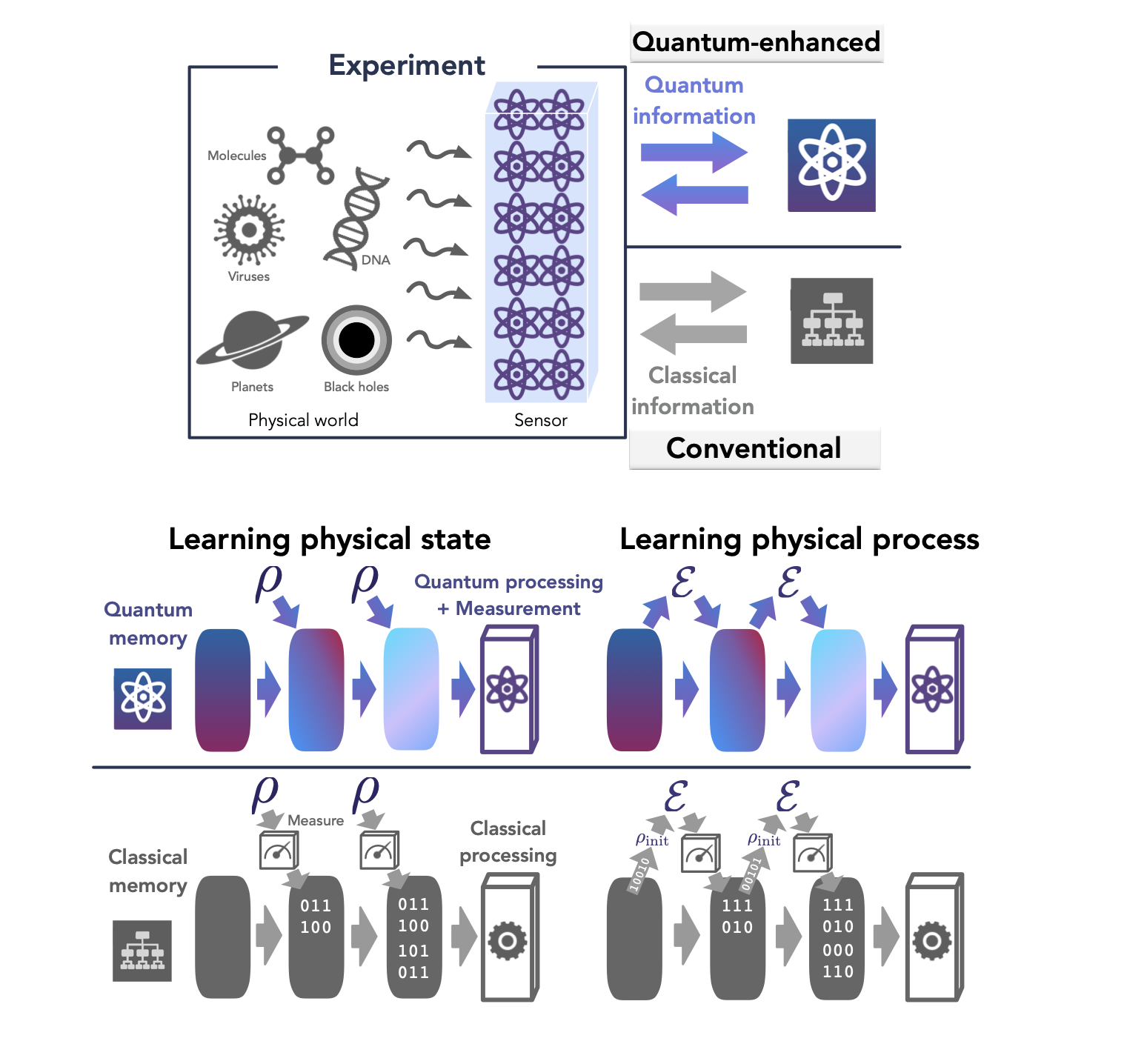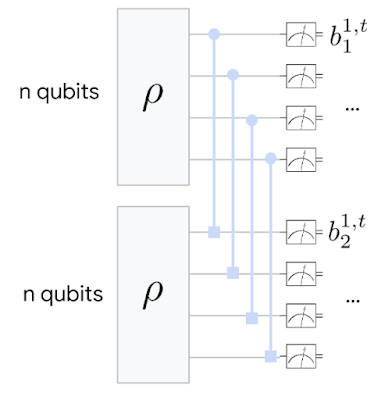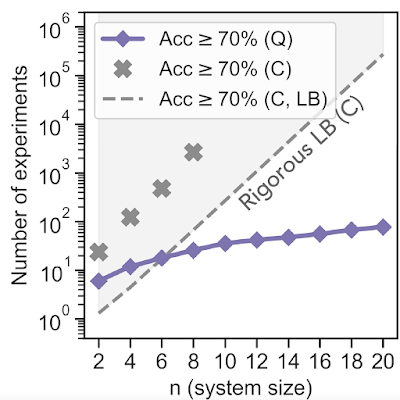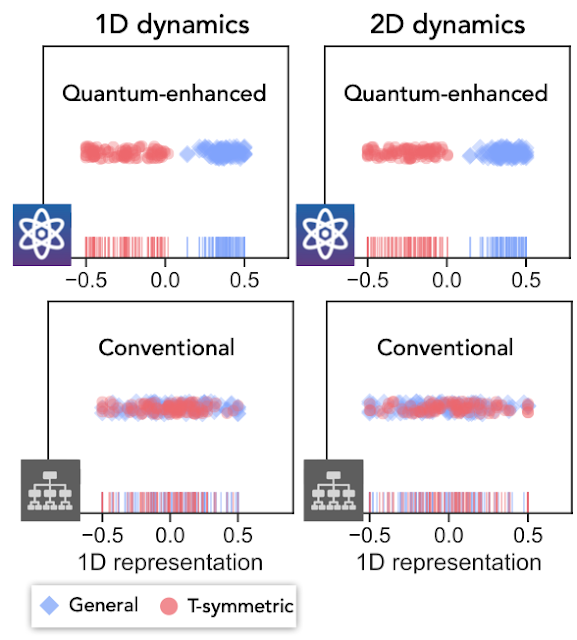# Quantum Advantage in Learning from Experiments

In efforts to learn about the quantum world, scientists face a big obstacle: their classical experience of the world. Whenever a quantum system is measured, the act of this measurement destroys the “quantumness” of the state. For example, if the quantum state is in a superposition of two locations, where it can seem to be in two places at the same time, once it is measured, it will randomly appear either ”here” or “there”, but not both. We only ever see the classical shadows cast by this strange quantum world.

A growing number of experiments are implementing machine learning (ML) algorithms to aid in analyzing data, but these have the same limitations as the people they aim to help: They can’t directly access and learn from quantum information. But what if there were a quantum machine learning algorithm that could directly interact with this quantum data?

In “Quantum Advantage in Learning from Experiments”, a collaboration with researchers at Caltech, Harvard, Berkeley, and Microsoft published in Science, we show that a quantum learning agent can perform exponentially better than a classical learning agent at many tasks. Using Google’s quantum computer, Sycamore, we demonstrate the tremendous advantage that a quantum machine learning (QML) algorithm has over the best possible classical algorithm. Unlike previous quantum advantage demonstrations, no advances in classical computing power could overcome this gap. This is the first demonstration of a provable exponential advantage in learning about quantum systems that is robust even on today's noisy hardware.

## Quantum Speedup

QML combines the best of both quantum computing and the lesser-known field of quantum sensing.

Quantum computers will likely offer exponential improvements over classical systems for certain problems, but to realize their potential, researchers first need to scale up the number of qubits and to improve quantum error correction. What’s more, the exponential speed-up over classical algorithms promised by quantum computers relies on a big, unproven assumption about so-called “complexity classes” of problems — namely, that the class of problems that can be solved on a quantum computer is larger than those that can be solved on a classical computer.. It seems like a reasonable assumption, and yet, no one has proven it. Until it's proven, every claim of quantum advantage will come with an asterisk: that it can do better than any known classical algorithm.

Quantum sensors, on the other hand, are already being used for some high-precision measurements and offer modest (and proven) advantages over classical sensors. Some quantum sensors work by exploiting quantum correlations between particles to extract more information about a system than it otherwise could have. For example, scientists can use a collection of N atoms to measure aspects of the atoms’ environment like the surrounding magnetic fields. Typically the sensitivity to the field that the atoms can measure scales with the square root of N. But if one uses quantum entanglement to create a complex web of correlations between the atoms, then one can improve the scaling to be proportional to N. But as with most quantum sensing protocols, this quadratic speed-up over classical sensors is the best one can ever do.

Enter QML, a technology that straddles the line between quantum computers and quantum sensors. QML algorithms make computations that are aided by quantum data. Instead of measuring the quantum state, a quantum computer can store quantum data and implement a QML algorithm to process the data without collapsing it. And when this data is limited, a QML algorithm can squeeze exponentially more information out of each piece it receives when considering particular tasks.Comparison of a classical machine learning algorithm and a quantum machine learning algorithm. The classical machine learning algorithm measures a quantum system, then performs classical computations on the classical data it acquires to learn about the system. The quantum machine learning algorithm, on the other hand, interacts with the quantum states produced by the system, giving it a quantum advantage over the CML.

To see how a QML algorithm works, it’s useful to contrast with a standard quantum experiment. If a scientist wants to learn about a quantum system, they might send in a quantum probe, such as an atom or other quantum object whose state is sensitive to the system of interest, let it interact with the system, then measure the probe. They can then design new experiments or make predictions based on the outcome of the measurements. Classical machine learning (CML) algorithms follow the same process using an ML model, but the operating principle is the same — it’s a classical device processing classical information.

A QML algorithm instead uses an artificial “quantum learner.” After the quantum learner sends in a probe to interact with the system, it can choose to store the quantum state rather than measure it. Herein lies the power of QML. It can collect multiple copies of these quantum probes, then entangle them to learn more about the system faster.

Suppose, for example, the system of interest produces a quantum superposition state probabilistically by sampling from some distribution of possible states. Each state is composed of n quantum bits, or qubits, where each is a superposition of “0” and “1” — all learners are allowed to know the generic form of the state, but must learn its details.

In a standard experiment, where only classical data is accessible, every measurement provides a snapshot of the distribution of quantum states, but since it’s only a sample, it is necessary to measure many copies of the state to reconstruct it. In fact, it will take on the order of 2n copies.

A QML agent is more clever. By saving a copy of the n-qubit state, then entangling it with the next copy that comes along, it can learn about the global quantum state more quickly, giving a better idea of what the state looks like sooner.Basic schematic of the QML algorithm. Two copies of a quantum state are saved, then a “Bell measurement” is performed, where each pair is entangled and their correlations measured.

The classical reconstruction is like trying to find an image hiding in a sea of noisy pixels — it could take a very long time to average-out all the noise to know what the image is representing. The quantum reconstruction, on the other hand, uses quantum mechanics to isolate the true image faster by looking for correlations between two different images at once.

## Results

To better understand the power of QML, we first looked at three different learning tasks and theoretically proved that in each case, the quantum learning agent would do exponentially better than the classical learning agent. Each task was related to the example given above:

1. Learning about incompatible observables of the quantum state — i.e., observables that cannot be simultaneously known to arbitrary precision due to the Heisenberg uncertainty principle, like position and momentum. But we showed that this limit can be overcome by entangling multiple copies of a state.
2. Learning about the dominant components of the quantum state. When noise is present, it can disturb the quantum state. But typically the “principal component” — the part of the superposition with the highest probability — is robust to this noise, so we can still glean information about the original state by finding this dominant part.
3. Learning about a physical process that acts on a quantum system or probe. Sometimes the state itself is not the object of interest, but a physical process that evolves this state is. We can learn about various fields and interactions by analyzing the evolution of a state over time.

In addition to the theoretical work, we ran some proof-of-principle experiments on the Sycamore quantum processor. We started by implementing a QML algorithm to perform the first task. We fed an unknown quantum mixed state to the algorithm, then asked which of two observables of the state was larger. After training the neural network with simulation data, we found that the quantum learning agent needed exponentially fewer experiments to reach a prediction accuracy of 70% — equating to 10,000 times fewer measurements when the system size was 20 qubits. The total number of qubits used was 40 since two copies were stored at once.Experimental comparison of QML vs. CML algorithms for predicting a quantum state’s observables. While the number of experiments needed to achieve 70% accuracy with a CML algorithm (“C” above) grows exponentially with the size of the quantum state n, the number of experiments the QML algorithm (“Q”) needs is only linear in n. The dashed line labeled “Rigorous LB (C)” represents the theoretical lower bound (LB) — the best possible performance — of a classical machine learning algorithm.

In a second experiment, relating to the task 3 above, we had the algorithm learn about the symmetry of an operator that evolves the quantum state of their qubits. In particular, if a quantum state might undergo evolution that is either totally random or random but also time-reversal symmetric, it can be difficult for a classical learner to tell the difference. In this task, the QML algorithm can separate the operators into two distinct categories, representing two different symmetry classes, while the CML algorithm fails outright. The QML algorithm was completely unsupervised, so this gives us hope that the approach could be used to discover new phenomena without needing to know the right answer beforehand.Experimental comparison of QML vs. CML algorithms for predicting the symmetry class of an operator. While QML successfully separates the two symmetry classes, the CML fails to accomplish the task.

## Conclusion

This experimental work represents the first demonstrated exponential advantage in quantum machine learning. And, distinct from a computational advantage, when limiting the number of samples from the quantum state, this type of quantum learning advantage cannot be challenged, even by unlimited classical computing resources.

So far, the technique has only been used in a contrived, “proof-of-principle” experiment, where the quantum state is deliberately produced and the researchers pretend not to know what it is. To use these techniques to make quantum-enhanced measurements in a real experiment, we’ll first need to work on current quantum sensor technology and methods to faithfully transfer quantum states to a quantum computer. But the fact that today’s quantum computers can already process this information to squeeze out an exponential advantage in learning bodes well for the future of quantum machine learning.

## Acknowledgements

We would like to thank our Quantum Science Communicator Katherine McCormick for writing this blog post. Images reprinted with permission from Huang et al., Science, Vol 376:1182 (2022).